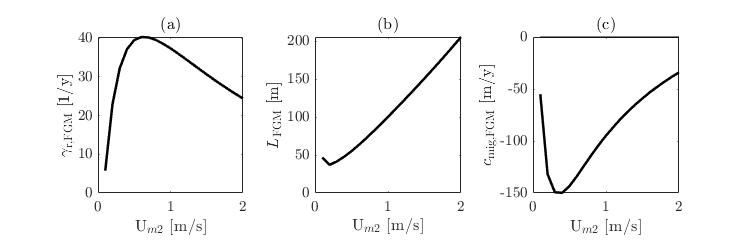D.S. Maas1*, W.M. van der Sande1, P.C. Roos1, S.J.M.H. Hulscher1

1 University of Twente

*corresponding author: This email address is being protected from spambots. You need JavaScript enabled to view it.

Introduction

All over the world, large-scale rhythmic bedforms can be found, one example being estuarine sand dunes. Estuarine sand dunes are influenced by both marine and fluvial processes. But they are also affected by estuarine-specific processes, like gravitation circulation. To understand the dynamics of the development of estuarine sand dunes, Van der Sande et al. (2021) developed a linear process-based model. This process-based model describes the initial development of estuarine sand dunes from a flat bed, and is based on shallow water equations forced by tide, river and a longitudinal salinity gradient. They choose their parameter settings to represent the Gironde Estuary. However, these parameters vary for different estuaries and locations within an estuary. Here, we investigate how the model parameters influence the characteristics of the Fastest Growing Mode (FGM), being growth rate, wavelength and migration rate.

Methods and results

We divide the parameters in two types: environmental parameters (water depth, depth-averaged M2 tidal velocity amplitude, depth-averaged river flow velocity, grain size and salinity gradient) and model parameters (drag coefficient, slip parameter, slope correction factor in the sediment equation and the bed load exponent). This is done to discern between natural variation and model uncertainty. The second step is to perform a one factor at the time (OFAT) sensitivity analysis.. This analysis shows the influence of different environmental and model parameters on the characteristics of the FGM. The range in each parameter is set based on a literature review. For example, the depth-averaged M2 tidal velocity amplitude ranges between 0.1 and 2 m/s, the effect of this change on the predicted sand dunes is presented in Figure 1. Thirdly, we investigate the combined sensitivity of the environmental parameter ranges and the model parameter ranges on the characteristics of the FGM. Lastly, we apply the model to explain variability between two cases: the Elbe and Scheldt estuary and the original used Gironde estuary .Figure 1: The effects of the depth-averaged M2 tidal velocity amplitude changes on the FGM properties. a) growth rate, b) wavelength, c) migration rate. This figure is an example of one of the OFAT analyses..

References

van der Sande, W. M., Roos, P. C., Gerkema, T., & Hulscher, S. J. M. H. (2021). Gravitational circulation as driver of upstream migration of estuarine sand dunes. Geophysical Research Letters, 48, e2021GL093337. https://doi.org/10.1029/2021GL093337